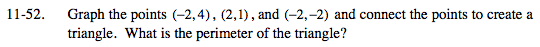### Home > MC2 > Chapter 11 > Lesson 11.2.3 > Problem11-52

11-52.Divide the triangle into two right triangles. Then use the graph to find some of the missing lengths.

Notice that the two triangles are similar. Find the hypotenuse of one of the triangles and that length will also be the length of the other hypotenuse. Use the Pythagorean Theorem.

Now add up all the sides to get the perimeter.

3² + 4² = hypotenuse²
25 = hypotenuse²

$\sqrt{25}=\sqrt{\text{hypotenuse}}$

hypotenuse = 5

(1, 6), (5, 3), and (1, 0)

See part (a).

Use the eTool below to graph the points and create a triangle.
Click the link at right for the full version of the eTool: MC2 11-52 HW eTool